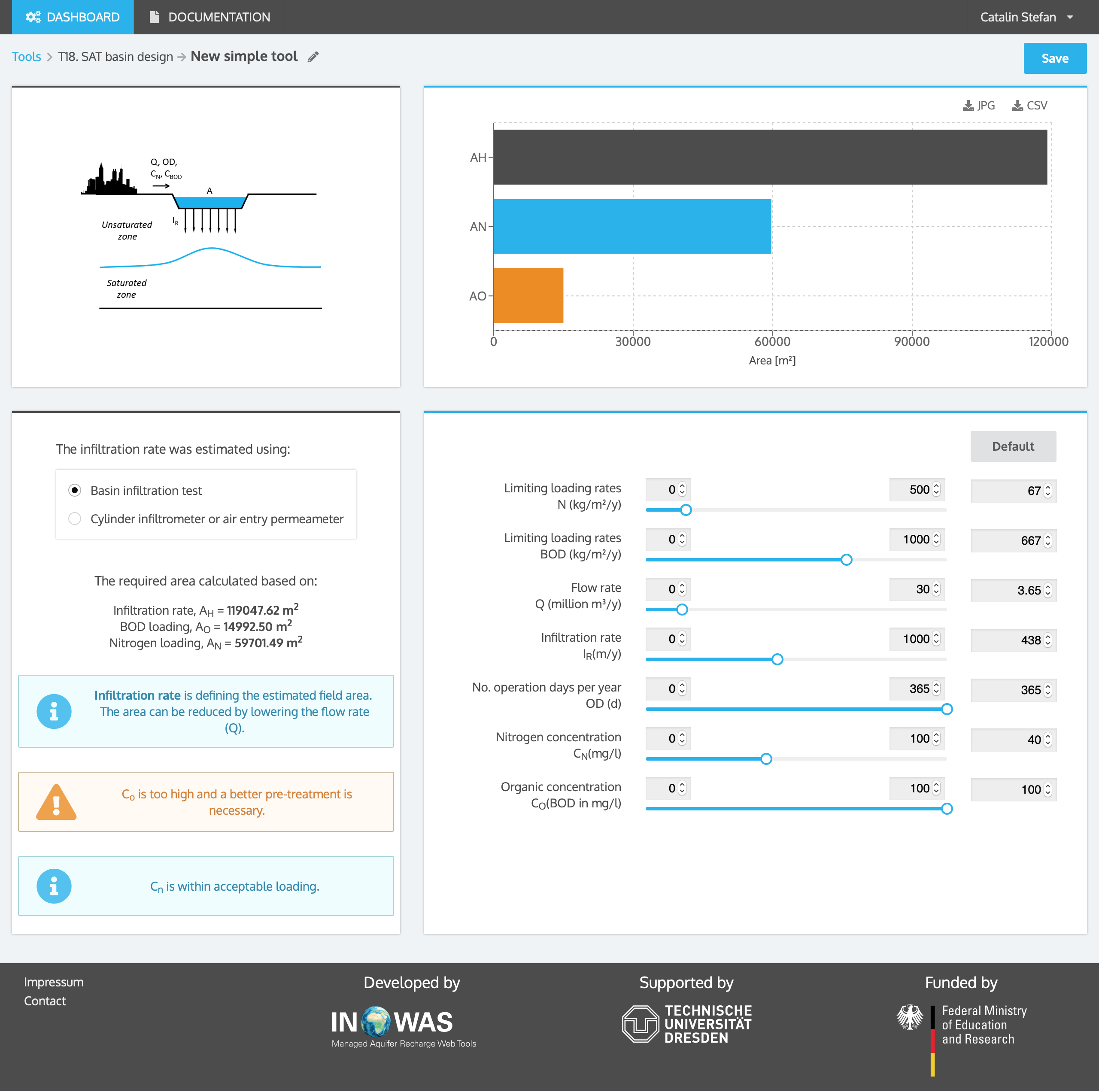# T18. SAT Basin Design

The tool contains empirical equations that can be used to design a typical soil aquifer treatment (SAT) system including hydraulic loading rates and land requirements under consideration of hydraulic characteristics of the soil and water quality such as nitrogen loading and organic loading respectively water quality requirements. The calculations are based on Crites (2014).

The hydraulic loading rate (HLR) defines the rate water is infiltrated per year and per square meter. It is the most important and critical design parameter for planning infiltration basins. The hydraulic loading rate is a function of the site-specific hydraulic capacity dependent on soil texture and bulk density. In some cases the nitrogen or organic loading can control the land required for infiltration; however, the limiting design factor for SAT systems is usually the infiltration/percolation rate.

The hydraulic loading rate used for designing the SAT system is the measured clean water infiltration rate multiplied by an adjustment factor which is depending on the procedure used for measuring the infiltration rate, on the variation in infiltration rate over the site and on the conservatism of the designer (eq. 1).

 (eq. 1)

where

 HLR = Hydraulic loading rate [LT-1] IR = Measured clean water infiltration rate [LT-1] af = Adjustment factor [-]

Adjustment factors account for the intermittent loading and drying in an SAT system and the long-term decrease in infiltration rates due to clogging processes as a result of wastewater loadings. Typical adjustment factors for different field tests are presented in the table below:

 Percent of measured infiltration rate values Test procedure Conservative range Less conservative range Basin infiltration test 5-7% 8-10% Cylinder infiltrometer or air entry permeameter 1-2% 2-4%

 (eq. 2)

where

 NLR = Nitrogen loading rate [ML-2T-1] HLR = Hydraulic loading rate [LT-1] CN = Nitrogen concentration in water [ML-1] D = Number of operating days per year [T]

 (eq. 3)

where

 OLR = Organic loading rate [ML-2T-1] HLR = Hydraulic loading rate [LT-1] CO = Concentration of organic matter in water expressed in biological oxygen demand (BOD) [ML-1] D = Number of operating days per year [T]

## Field area, A

The area required for an SAT system is calculated based on the hydraulic loading rate and the annual water flow rate into the system (eq. 4):

 (eq. 4)

where

 AH = Field area [L2] Q = Annual water flow rate into basin [L3T-1] HLR = Hydraulic loading rate [LT-1]

In some cases, the required area can be decided by the high concentration of nitrogen or organic matter in the infiltration water. In theses cases, the respective areas ANand AOneed to be calculated based on nitrogen and organic matter concentration and the corresponding limiting loading rates LLRNand LLRO (eq. 5 and 6). The final required area is then the maximum area between AH, ANand AO.

 (eq. 5)
 (eq. 6)

where

 AN, AO = Field area  [L2] CN, CO = Nitrogen / organic matter concentration (expressed as BOD) in the water [ML-1] Q = Annual water flow rate into basin [L3T-1] LLRN, LLRO = Limiting nitrogen or organic loading rate [ML-2T-1]

The online calculator needs user-supplied values for infiltration rate (IR) measured by infiltration test, annual water flow to the basin (Q), nitrogen and organic matter concentration (CN/CO) in the water flowing into the basin.

### Example